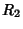At each point on a given a 2-D Surface, there are two ``principal'' Radii of Curvature. The larger is denoted, and the smaller. The ``principal directions'' corresponding to the principal radii of curvature are Perpendicular to one another. In other words, the surface normal planes at the point and in the principal directions are Perpendicular to one another, and both are Perpendicular to the surface tangent plane at the point.